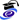# Marco CaliariPosition
Associate Professor
MAT/08 - NUMERICAL ANALYSIS
Research sector (ERC)
PE1_17 - Numerical analysis

Office
Ca' Vignal 2,  Floor 2,  Room 3
Telephone
+39 045 802 7904
Fax
+39 045 802 7068
E-mail
marcocaliariunivritPersonal web page
http://profs.scienze.univr.it/caliari

#### Office Hours

Tuesday, Hours 2:30 PM - 4:30 PM,   Ca' Vignal 2, Floor 2, room 3

Curriculum
•Curriculum Vitae   (pdf, it, 105 KB, 29/06/17)
•Curriculum Vitae (English)   (pdf, en, 88 KB, 29/06/17)

Marco Caliari si occupa di:
• approssimazione dell'esponenziale di matrice e della sua azione attaverso polinomi con applicazione agli integratori esponenziali
• soluzione di equazioni di Schrödinger non lineari per la simulazione di condensati di Bose-Einstein e superfluidi attraverso metodi di splitting
Le sue pubblicazioni si collocano prevalentemente sulle riviste internazionali dell'area dell'analisi numerica.

#### Modules

Modules running in the period selected: 53.
Click on the module to see the timetable and course details.

Course Name Total credits Online Teacher credits Modules offered by this teacher
Bachelor's degree in Applied Mathematics Numerical analysis II with laboratory (2019/2020)   6    (Laboratorio)
Bachelor's degree in Applied Mathematics Numerical analysis I with laboratory (2019/2020)   6    (Laboratorio)
Bachelor's degree in Applied Mathematics Numerical methods for differential equations (2019/2020)   6
Master's degree in Mathematics Numerical methods for partial differential equations (2019/2020)   6
Master's degree in Mathematics Advanced numerical analysis II (2018/2019)   6(Teoria)
Bachelor's degree in Applied Mathematics Numerical analysis II with laboratory (2018/2019)   6    (Laboratorio)
Bachelor's degree in Applied Mathematics Numerical analysis I with laboratory (2018/2019)   6    (Laboratorio)
Bachelor's degree in Applied Mathematics Numerical methods for differential equations (2018/2019)   6
Master's degree in Mathematics Scientific computing (seminar course) (2018/2019)   6
Bachelor's degree in Applied Mathematics Stochastic systems (2018/2019)   6(Esercitazioni)
Master's degree in Mathematics Advanced numerical analysis II (2017/2018)   6Bachelor's degree in Applied Mathematics Numerical analysis II with laboratory (2017/2018)   6
Bachelor's degree in Applied Mathematics Numerical methods for differential equations (2017/2018)   6
Master's degree in Mathematics Scientific computing (seminar course) (2017/2018)   6
Bachelor's degree in Applied Mathematics Stochastic systems (2017/2018)   6Master's degree in Mathematics Advanced numerical analysis II (2016/2017)   6
Bachelor's degree in Applied Mathematics Numerical methods for differential equations (2016/2017)   6
Master's degree in Mathematics Scientific computing (seminar course) (2016/2017)   6Bachelor's degree in Applied Mathematics Stochastic systems (2016/2017)   6
Master's degree in Mathematics Advanced numerical analysis II (2015/2016)   6
Bachelor's degree in Applied Mathematics Numerical methods for differential equations (2015/2016)   6
Master's degree in Mathematics Research and modelling seminar (seminar course) (2015/2016)   6
Bachelor's degree in Applied Mathematics Stochastic systems (2015/2016)   6    (esercitazioni)
Master's degree in Mathematics Advanced numerical analysis II (2014/2015)   6
Bachelor's degree in Applied Mathematics Numerical methods for differential equations (2014/2015)   6    (Teoria)
(Laboratorio)
Master's degree in Mathematics Scientific computing (seminar course) (2014/2015)   6
Bachelor's degree in Applied Mathematics Stochastic systems (2014/2015)   6    (Esercitazioni)
Master's degree in Mathematics Advanced numerical analysis (2013/2014)   12    (Laboratorio)
Bachelor's degree in Applied Mathematics Numerical methods for differential equations (2013/2014)   6    (Teoria)
(Esercitazioni)
Bachelor's degree in Applied Mathematics Stochastic systems (2013/2014)   6    (Esercitazioni)
Master's degree in Mathematics Advanced numerical analysis (2012/2013)   12    (Laboratorio)
Bachelor's degree in Applied Mathematics Numerical methods for differential equations (2012/2013)   6    (Esercitazioni)
(Teoria)
Master's degree in Mathematics Scientific computing (seminar course) (2012/2013)   6
Bachelor's degree in Applied Mathematics Stochastic systems (2012/2013)   6    (Esercitazioni)
Bachelor's degree in Applied Mathematics Numerical methods for differential equations (2011/2012)   6    (Teoria)
(Esercitazioni)
Master's degree in Mathematics Scientific computing (2011/2012)   6
Bachelor's degree in Applied Mathematics Stochastic systems (2011/2012)   6    (Esercitazioni)
Bachelor's degree in Applied Mathematics Numerical methods for differential equations (2010/2011)   6(Teoria)
Master's degree in Mathematics Scientific computing (2010/2011)   6
Bachelor's degree in Applied Mathematics Stochastic systems (2010/2011)   6    (Esercitazioni)
Master's degree in Mathematics Metodi numerici avanzati per le equazioni differenziali alle derivate parziali (2010/2011)   6
Bachelor's degree in Applied Mathematics Numerical methods for differential equations (2009/2010)   6
Bachelor's degree in Applied Mathematics Stochastic systems (2009/2010)   6    (Laboratorio)
Degree in Applied Mathematics (until a.y. 2008/2009) Numerical Analysis (2008/2009)   8    Laboratorio
Degree in Applied Mathematics (until a.y. 2008/2009) Numerical methods for differential equations (2008/2009)   6    Laboratorio
Degree in Applied Mathematics (until a.y. 2008/2009) Stochastic systems (2008/2009)   6    Laboratorio
Degree in Applied Mathematics (until a.y. 2008/2009) Basic Mathematics (2007/2008)   4
Degree in Applied Mathematics (until a.y. 2008/2009) Numerical Analysis (2007/2008)   8    Laboratorio
Degree in Applied Mathematics (until a.y. 2008/2009) Numerical methods for differential equations (2007/2008)   6    Laboratorio

#### Research groups

INdAM - Unità di Ricerca dell'Università di Verona
Questa pagina è dedicata all'unità di ricerca INdAM dell'Università di Verona.
Mathematics - applications and modelling
Skills
Topic Description Research area
Approximation of matrix exponential Polynomial approximation of matrix exponential by interpolation at special nodes. Mathematics - applications and modelling
Numerical analysis
Numerical approximation We implement algorithms to calculate a numerical approximation of a complicated function, defined either directly by an explicit formula or procedure or else, for example, defined indirectly as the solution of a differential equation of some type. Mathematics - applications and modelling
Numerical analysis
Partial differential equations, initial value and time-dependent initial-boundary value problems Solution of non-linear Schrödinger, mean-field and Boltzmann-type equations by pseudo-spectral or meshless methods in space and splitting methods in time. Mathematics - applications and modelling
Numerical analysis
Projects
Title Starting date
Approssimazione multivariata con basi polinomiali e radiali 2/29/12
Interpolazione multivariata con polinomi, RBF e altre basi e applicazioni (PRIN 2009) 7/15/11
Meshfree exponential integrators 1/1/11
Near Optimal Points for Multivariate Interpolation 1/1/10
Metodi numerici per equazioni di Schroedinger non lineari 2/9/09
Interpolazione ed Estrapolazione: nuovi algoritmi ed applicazioni 1/1/09
Interpolazione multivariata con polinomi e con funzioni radiali di base: analisi di stabilita`, implementazioni efficienti e applicazioni (PRIN 2007) 9/22/08
Modelli stocastici in dimensione finita e infinita e limiti di scala (PRIN 2004 biennale) 11/30/04